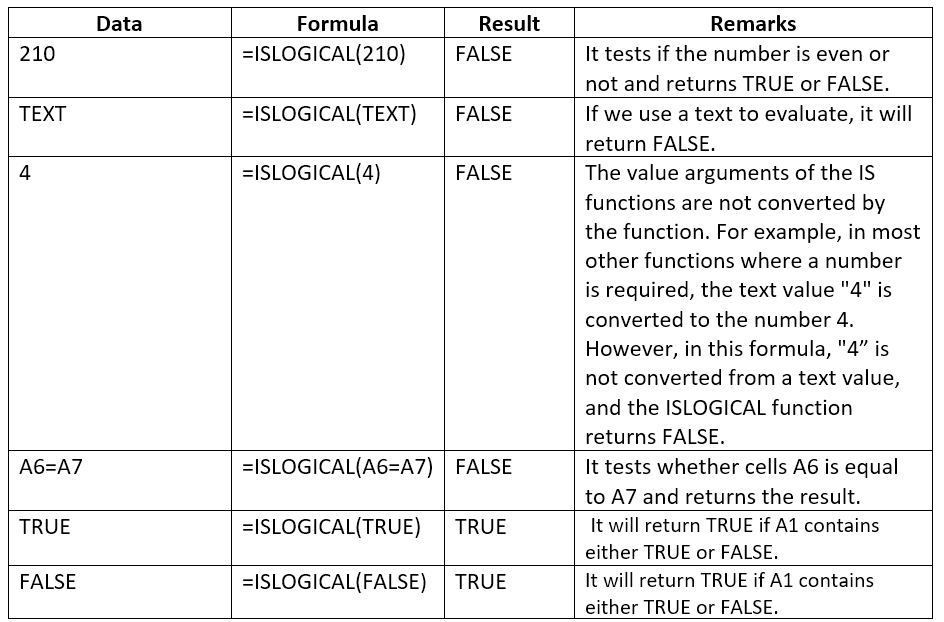# ISLOGICAL Function

Tests the value or expression provided and returns a logical value

## What is the ISLOGICAL Function?

The ISLOGICAL function is categorized underExcel Information functions. The function will test the value or expression provided and return a logical value. That is, it will evaluate the value or expression to provide a TRUE or FALSE result. It is part of a group of functions called the IS functions, which are commonly used to test the result of formulas for errors.

In financial analysis, we often deal with numbers. The ISLOGICAL function is useful for checking errors in our data or analysis where we used some formulas.

### Formula

=ISLOGICAL(value)

The ISLOGICAL function uses the following arguments:

1. Value (required argument) – This is the value we wish to test as logical or not. Value can be a blank (empty cell), error, logical, text, number, or reference value, or a name referring to any of these, that we want to test.

### How to use the ISLOGICAL Function in Excel?

To understand the uses of the ISLOGICAL function, let’s consider a few examples:

#### Example 1

Let’s see the results from the function when we provide the following data:### Things to remember about the ISLOGICAL Function:

1. The function returns FALSE for a blank cell.
2. 1 and 0 (zero) are not evaluated as TRUE and FALSE. However, you can use the AND function to evaluate 1 and 0 as TRUE and FALSE.
3. If the value provided us contains an error, ISLOGICAL will return a #N/A value.

Thanks for reading CFI’s guide to important Excel functions! By taking the time to learn and master these functions, you’ll significantly speed up your financial analysis. To learn more, check out CFI’s financial modeling courses, and these additional resources:

• Excel Functions for Finance